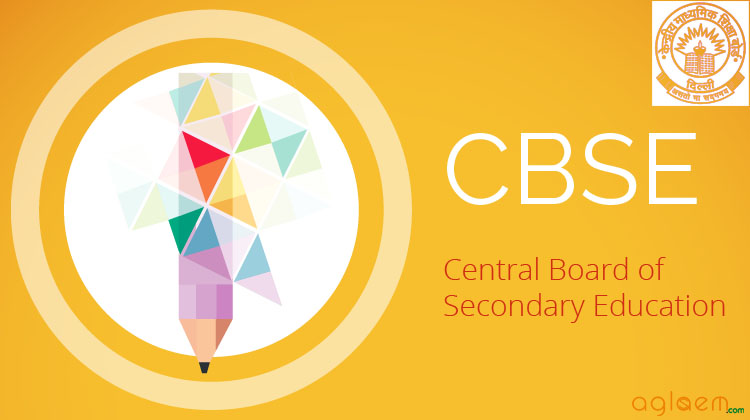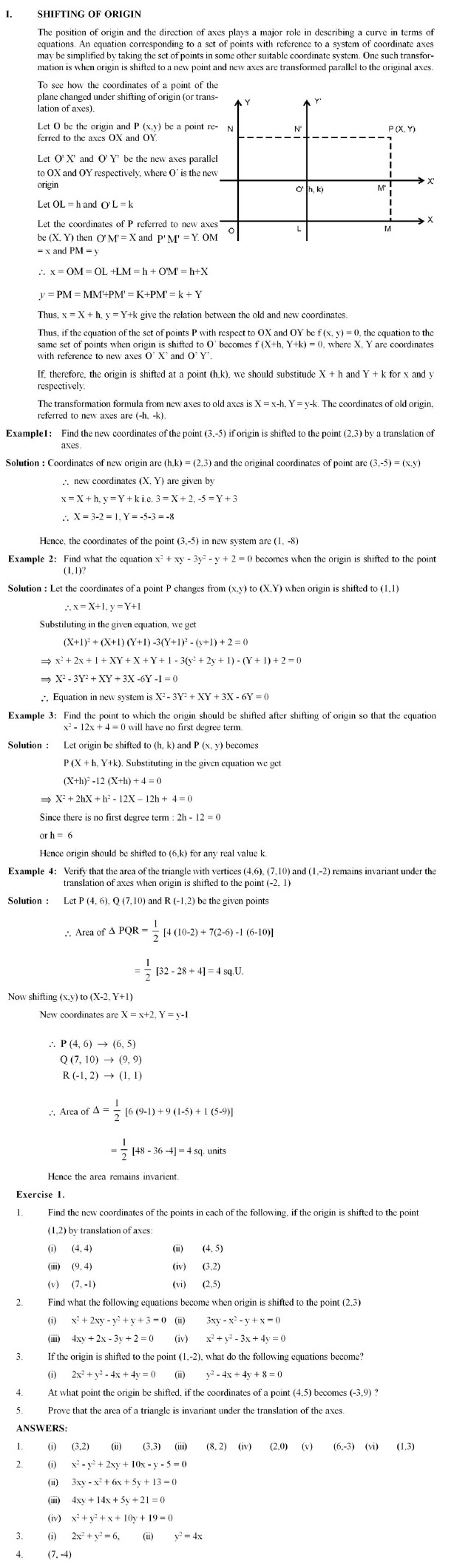## CBSE Class XI Supplementary Textual Material in Mathematics

Contents:

• Chapter 3:
• Trigonometric Functions
• Law of Sines and Law of Cosines
• Chapter 5:
• Complex Numbers and Quadratic Equations
• Square Root of a Complex Number
• Chapter 9:
• Straight Line
• Shifting of Origin
• Chapter 13:
• Limits and Derivative
• Some Important Limits

CBSE Class 11 Supplementary Textual Material in Mathematics is given below.

CHAPTER 3 – TRIGONOMETRIC FUNCTIONSCHAPTER 5 – COMPLEX NUMBERS AND QUADRATIC EQUATIONCHAPTER 9 – SEQUENCES AND SERIESCHAPTER 10 – STRAIGHT LINECHAPTER 13 – LIMITS AND DERIVATIVES## CBSE Class XII Supplementary Textual Material in Mathematics

Contents:

• Chapter 5:
• Continuity and Differentiation
• Derivatives of logeX and ex
• Chapter 7:
• Integrals
• Integrals of tyle ∫√(ax2 + bs + c) dx, ∫(px + q)√(ax2 + bs + c) dx
• Chapter 10:
• Vector Algebra
• Scaler Triple Product

CBSE Class 12 Supplementary Textual Material in Mathematics is given below.

CHAPTER 5 – CONTINUITY AND DIFFERENTIATIONCHAPTER 7 – INTEGRALSCHAPTER 10 – VECTOR ALGEBRA